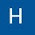## Trang

If you already make heavy use of Google Translate, this add-on can save a lot of time. Instead of having to open Google Translate in a new browser window, you can just highlight some text and translate it from inside the document.

After installing it, start it from the Add-ons menu. To translate your Docs content, let select some text, choose the languages and you’re all set then click the Translate button. You can then insert the translation back into your document.

1.the Translate+ was going white and glitching every time I hit "translate" and I had to exit and start again plus the "set language as default" thing doesn't work.

Like if you agree.<3<3<3<3<3<3

1.<3<3<3<3<3<3<3<3<3<3<3<3<3<3<3<3<3<3<3<3<3<3<3<3<3<3<3<3<3<3<3<3<3<3<3<3<3<3<3<3<3<3<3<3<3<3<3<3<3<3<3<3<3<3<3<3<3<3<3<3<3<3<3<3<3<3<3<3<3<3<3<3<3<3<3<3<3<3<3<3<3<3<3<3<3<3<3<3<3<3<3<3<3<3<3<3<3<3<3<3<3<3<3<3<3<3<3<3<3<3<3<3<3<3<3<3<3<3<3<3<3<3<3<3<3<3<3<3<3<3<3<3<3<3<3<3<3<3<3<3<3<3<3<3<3<3<3<3<3<3<3<3<3<3<3<3<3<3<3<3<3<3<3<3<3<3<3<3<3<3<3<3<3<3<3<3<3<3<3<3<3<3<3<3<3<3<3<3<3<3<3<3<3<3<3<3<3<3<3<3<3<3<3<3<3<3<3<3<3<3<3<3<3<3<3<3<3<3<3<3<3<3<3<3<3<3<3<3<3<3<3<3<3<3<3<3<3<3<3<3<3<3<3<3<3<3<3<3<3<3<3<3<3<3<3<3<3<3<3<3<3<3<3<3<3<3<3<3<3<3<3<3<3<3<3<3<3<3<3<3<3<3<3<3<3<3<3<3<3<3<3<3<3<3<3<3<3<3<3<3

2.the bug has been fixed

3.I think that Wing Ding should be added

2.NOPE<3<3<3<3<3<3<3<3<3<3<3<3<3<3<3<3<3<3<3<3<3<3<3<3<3<3<3<3<3<3<3<3<3<3<3<3<3<3<3<3<3<3<3<3<3<3<3<3<3<3<3<3<3<3<3<3<3<3<3<3<3<3<3<3<3<3<3<3<3<3<3<3<3<3<3<3<3<3<3<3<3<3<3<3<3<3<3<3<3<3<3<3<3<3<3<3<3<3<3<3<3<3<3<3<3<3<3<3<3<3<3<3<3<3<3<3<3<3<3<3<3<3<3<3<3<3<3<3<3<3<3<3<3<3<3<3<3<3<3<3<3<3<3<3<3<3<3<3<3<3<3<3<3<3<3<3<3<3<3<3<3<3<3<3<3<3<3<3<3<3<3<3<3<3<3<3<3<3<3<3<3<3<3<3<3<3<3<3<3<3<3<3<3<3<3<3<3<3<3<3<3<3<3<3<3<3<3<3<3<3<3<3<3<3<3<3<3<3<3<3<3<3<3<3<3<3<3<3<3<3<3<3<3<3<3<3<3<3<3<3<3<3<3<3<3<3<3<3<3<3<3<3<3<3<3<3<3<3<3<3<3<3<3<3<3<3<3<3<3<3<3<3<3<3<3<3<3<3<3<3<3<3<3<3<3<3<3<3<3<3<3<3<3<3<3<3<3<3<3<3

3.4.Is it possible to add an option "use informal language" for a target language, eg. German "Sie" (formal) vs. "du" as translation of English "you"?

5.6.7.This comment has been removed by the author.

8.Everytime I click on "Translate" the box goes blank.

9.it wont work.

10.start option is not showing

1.Same for me

11.Hi, I won't work for me, translate + box opens I select text and press translate, the box goes blank with just the top bar left. Shame it looks really good.

1.Same with me

2.same just the help button no start
I am sad, this was going to be a great help to me

12.Hi OK I got it working but I had to install Chrome I had been trying to use it in Firefox.

13.it doesn't work one bit on my brand new model laptop

14.lalalalalalonlalalalalalonlonlilonlonlonahiolalololololonalalolonlololollilonlon

15.this does not work cannot start the translation within the dropdown,

Why release something that is not functional

16.لم يكن لدية في هذا البرنامج ميغافون لكتابة الصوتيات

17.Сайт drive.google.com не позволяет установить соединение.
ERR_BLOCKED_BY_RESPONSE

What does that mean? How to fix it?

18.After Chrome's last update, Translate+ goes blank if I don't use for a few minutes, and need to reset it all over again.

19.ScriptError: Translation between the given languages is not currently supported.
isso é a mensagem que sempre recebo quando preciso de tradução, já a recebi em diversas ocasiões

20.d<ont have start button

1.21.the space of translation became small compared with before.
How can I make the space larger?

22.gkms_email
hamdikhalid43@gmail.com
subject_line
استعلامك المتعلّق بأدوات حقوق الطبع والنشر
Language
ar
IIILanguage
ar
country_code
YE
auto-helpcenter-id
95
auto-helpcenter-name
auto-internal-helpcenter-name
auto-full-url
auto-user-logged-in
true
auto-user-was-internal
false
IssueType
form-id

23.dont have start button

24.25.It stops working.

26.1.Can't update? me too

27.This comment has been removed by the author.

28.It doesn´t work well. So slow and I couldn´t translate any document.

29.Had been working fine until today. Now I got to 4th translation selection of the day (i.e. 4th word, not sentence or paragraph) and I get "ScriptError: Service invoked too many times for one day: translate." It won't work on one word or a group of words. Please advise.

30.Let's dye this world,
Even I can not grap this sword,
All I need is getting strong,
We can catch the game

31.استلام دفعات ادسينس"حوالة مصرفيه"برقم برقم

Yemen/Taizبطاقةهويتي04010308415الوطنيه

32.bet365 casino and sportsbook - Jordan13 Sports
Bet365 is a privately 메이저 안전 놀이터 held company based air jordan 18 retro red free shipping in London, England. The firm offers sports betting, bingo, casino, how can i get air jordan 18 retro toro mens sneakers poker, bingo and air jordan 18 retro men blue to my site gambling. Bet365 is air jordan 18 retro yellow

33.I would like to thank you for this app. It's really very helpful. I use it every day in my work. However, I would like to suggest you to make some update to make it possible after pushing the button "Translate" to move to "Insert into Doc" button by pressing the "Tab". It would be great to have such an improvement. Thank you in advance. Great job.